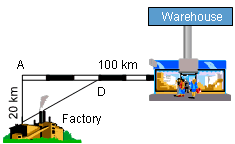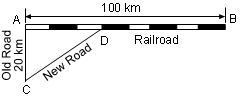Ch 4. The Mean Value Theorem Multimedia Engineering Math Maximum & Minimum Rolle'sTheorem Mean ValueTheorem MonotonicFunctions First DerivativeTest Concavity &Inflection SecondDerivative Test
 Chapter 1. Limits 2. Derivatives I 3. Derivatives II 4. Mean Value 5. Curve Sketching 6. Integrals 7. Inverse Functions 8. Integration Tech. 9. Integrate App. 10. Parametric Eqs. 11. Polar Coord. 12. Series Appendix Basic Math Units Search eBooks Dynamics Fluids Math Mechanics Statics Thermodynamics Author(s): Hengzhong Wen Chean Chin Ngo Meirong Huang Kurt Gramoll ©Kurt GramollMATHEMATICS - CASE STUDY IntroductionProblem Description A factory that is located 20 km from a railway would like to reduce the rransportation costs by building a new road to the rail line. This will, however, increase the road transprotation costs to the railroad, but reduce the railroad costs. Where should the new rail link, D be located so that the transportation costs are the minimum. What is known: The length of the old railway, AB, is 100 km. The old road distance from the factory to the railway is 20 km as shown in the diagram. The cost to ship by rail verses road is 3 to 5. Questions Where should the rai link, D, be built so that the transportation costs are the lowest?Simplified Problem Diagram Approach The total transportation cost is the sum of the railroad and road costs. The minimum transportation cost may occur when the rail link, D, is located at A, B or somewhere between A and B.